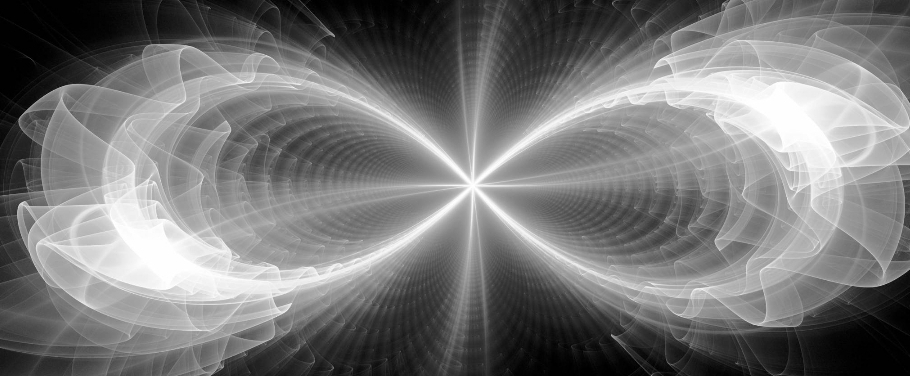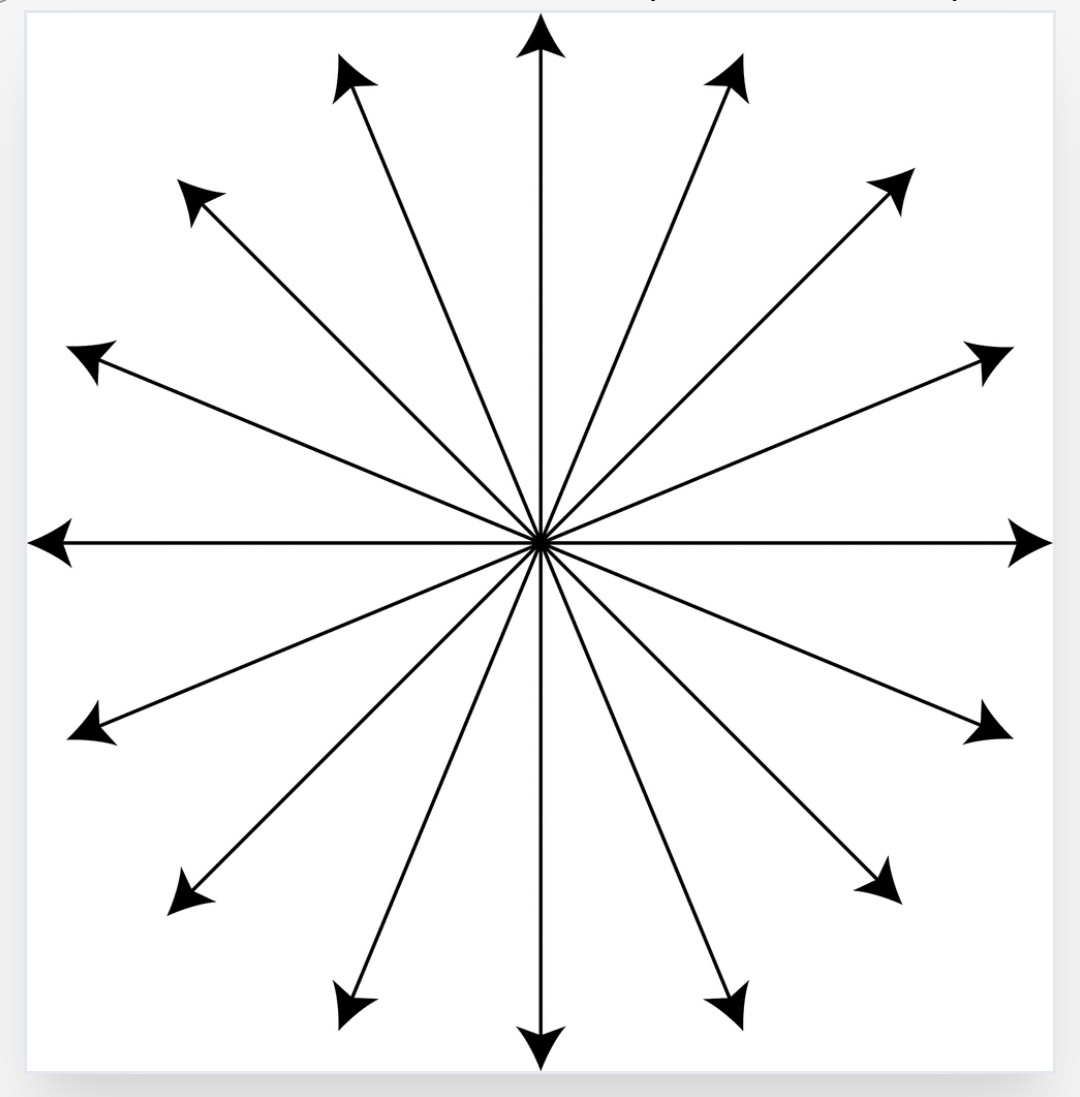Jan. 16, 2021

# Infinity's LimitsInfinity’s Center

12/12/17(edited 1/15/21)

The number 1 is the first counting number and there is an infinite number of numbers between the two nearest whole numbers on either side e.g. 0……….1……….2.  Based on our concept of infinity, you could never truly count from 0 to 1 or from 1 to 2 because of the infinity between each digit.

All counting numbers after 1, are a collection of 1’s.  15,736 is really a shorthand way of expressing 15,736 1’s!!  All counting numbers are based on the foundational number 1.  Even the whole number 0 is defined by the first counting number 1 because without 1 there is no concept of what 0 is just as we understand what temperature is because of heat, we understand what evil is because of good, etc.  The number 1, like good and heat, define all other concepts related to them.

This theme is seen throughout creation when we consider that all life begins when 1 cell(sperm) unites with 1 cell(egg) to create a 1 cell(zygote) or when 1 cell divides into 2 cells(1+1).  1 as the foundation of all things is consistent with monotheism as well. The concept of 1 Creator giving rise to all is as foundational as 1 being the foundation from which all numbers flow.

The number system is just one of the many constructs that we use to try and help us relate to that which is beyond comprehension.  This centrality of 1 is a proof that 1 is the center of infinity.

Notice in Diagram A, 1 point is the center of infinity in any direction taken from the central point!!!  The Alpha and Omega are that central point.

*any direction you go in from 1 is infinity!!!

Diagram A:Limits of Infinity

Infinity is an abstract concept that describes something that is without boundaries, innumerable, beyond comprehension, immeasurable.  This concept is yet another attempt to comprehend that which is beyond comprehension.  The fact of the matter is infinity is limited, it starts and stops.  Look at Diagram B, here we see the universal symbol for infinity encompassed on each side by vertical bars which represent the limits of infinity.  Our minds cannot comprehend even a tiny fraction of what infinity means, yet it is limited.  Notice outside the vertical limit bars, on one end is the Alpha(𝞐) symbol, the true beginning of all things and on the other end the Omega symbol(Ω), the true end.

As you can see infinity is found within the Alpha and the Omega which makes it totally limited to that from which it is derived, the Alpha and Omega.  Furthermore, the Alpha and Omega are one in the same, therefore infinity as we know it is limited to 1!!!!

Diagram B.

𝞐IIΩ